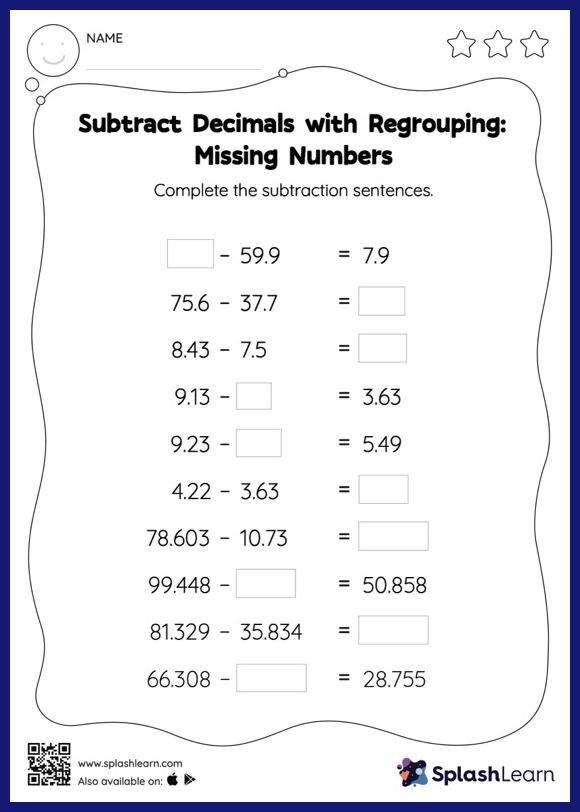# Subtract Decimals with Regrouping: Missing Numbers Worksheet

Home > Subtract Decimals with Regrouping: Missing NumbersSubtract decimals with regrouping worksheet is a great way to help learners become fluent with the concept of subtraction. While subtracting decimals, students use the relationship between addition and subtraction to find the missing number. They also need to regroup numbers in subtract decimals with regrouping worksheet utilizing the relationship between ones, tenth, hundredth, thousandth, etc., To get to the result.